Thermodynamic Potential NAT Level – 2

# Thermodynamic Potential NAT Level – 2

Test Description

## 10 Questions MCQ Test Topic wise Tests for IIT JAM Physics | Thermodynamic Potential NAT Level – 2

Thermodynamic Potential NAT Level – 2 for IIT JAM 2023 is part of Topic wise Tests for IIT JAM Physics preparation. The Thermodynamic Potential NAT Level – 2 questions and answers have been prepared according to the IIT JAM exam syllabus.The Thermodynamic Potential NAT Level – 2 MCQs are made for IIT JAM 2023 Exam. Find important definitions, questions, notes, meanings, examples, exercises, MCQs and online tests for Thermodynamic Potential NAT Level – 2 below.
Solutions of Thermodynamic Potential NAT Level – 2 questions in English are available as part of our Topic wise Tests for IIT JAM Physics for IIT JAM & Thermodynamic Potential NAT Level – 2 solutions in Hindi for Topic wise Tests for IIT JAM Physics course. Download more important topics, notes, lectures and mock test series for IIT JAM Exam by signing up for free. Attempt Thermodynamic Potential NAT Level – 2 | 10 questions in 45 minutes | Mock test for IIT JAM preparation | Free important questions MCQ to study Topic wise Tests for IIT JAM Physics for IIT JAM Exam | Download free PDF with solutions
 1 Crore+ students have signed up on EduRev. Have you?
*Answer can only contain numeric values
Thermodynamic Potential NAT Level – 2 - Question 1

### For black body radiation, entropy varies with temperature as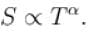Find the value of α?

Detailed Solution for Thermodynamic Potential NAT Level – 2 - Question 1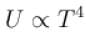(Stefan-Boltzman's lane)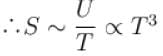*Answer can only contain numeric values
Thermodynamic Potential NAT Level – 2 - Question 2

### For a fermi gas (in 8 dimension) value of the ratio Cp/CV is?

Detailed Solution for Thermodynamic Potential NAT Level – 2 - Question 2

Average energy of Fermious is given by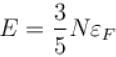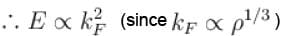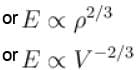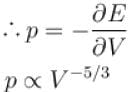pV5/3= constant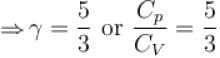*Answer can only contain numeric values
Thermodynamic Potential NAT Level – 2 - Question 3

### A quantity of heat ΔH is transferred from a large heat reservoir at temperature T to another large heat reservoir at temperature T/2. The heat reservoir have such large capacities that there is no observable change in true temperature. The ratio of the entropy change of the entire system to the entropy change in heat reservoir at temperature T/2 is?

Detailed Solution for Thermodynamic Potential NAT Level – 2 - Question 3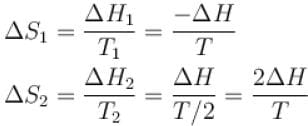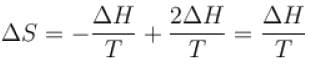Now,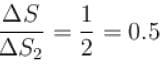*Answer can only contain numeric values
Thermodynamic Potential NAT Level – 2 - Question 4

At high temperature, entropy of a spin half system is equal to NkB time the natural logarithm of what number?

Detailed Solution for Thermodynamic Potential NAT Level – 2 - Question 4

zcosh(βμH) = 1 and μHβ[tanh(βμH)] = 0
∴ Entropy for spin 1/2 system which given by
S = Nk[ln{2cosh(βμH)} – βμHtanh(βμH)]
Reduces to S = NkBln 2

*Answer can only contain numeric values
Thermodynamic Potential NAT Level – 2 - Question 5

Virial of a system is defined as V = Σqipi , where qi is a generalized coordinate and pi conjugate momentum. Value of V for a system consisting of N particles at temperature T is equal to aNkBT. Find the value of constant a?

Detailed Solution for Thermodynamic Potential NAT Level – 2 - Question 5

Virial theorem states that
V = –2k·E
Since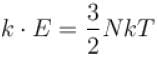⇒ V = –3NkT
⇒ a = –3

*Answer can only contain numeric values
Thermodynamic Potential NAT Level – 2 - Question 6

Entropy of a system of N classical harmonic oscillator having frequency ω and at temperature T is given by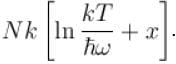Find the value of constant x?

Detailed Solution for Thermodynamic Potential NAT Level – 2 - Question 6

For Harmonic oscillator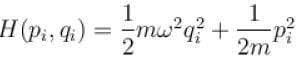∴ Single Oscillator partition function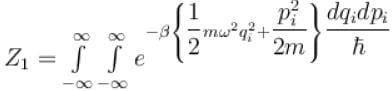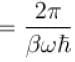∴ N-oscillator partition function
Z = (Z1 )N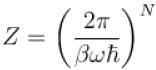∴ Helmholtz free energy function
F = –kTlnZ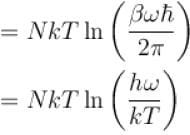∴ Entropy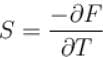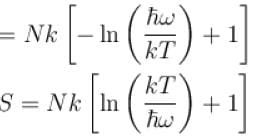∴ x = 1

*Answer can only contain numeric values
Thermodynamic Potential NAT Level – 2 - Question 7

The temperature of a cavity of fixed volume is doubled. Its energy increases by α times whereas number of photons increase by β times. Then calculate α/β?

Detailed Solution for Thermodynamic Potential NAT Level – 2 - Question 7

Energy of blackbody radiation αT4 and equilibrium number of photons in cavity αT3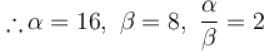*Answer can only contain numeric values
Thermodynamic Potential NAT Level – 2 - Question 8

For non interacting fermions entropy value with temperature for T → 0 as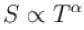. Find the value of α.

Detailed Solution for Thermodynamic Potential NAT Level – 2 - Question 8

For T → 0 internal energy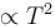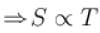⇒ α = 1

*Answer can only contain numeric values
Thermodynamic Potential NAT Level – 2 - Question 9

The mean internal energy of a one-dimensional harmonic oscillator in equilibrium with a heat bath of temperature T is in the units of kBT.

Detailed Solution for Thermodynamic Potential NAT Level – 2 - Question 9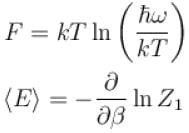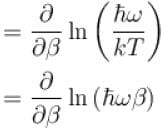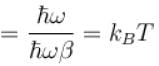*Answer can only contain numeric values
Thermodynamic Potential NAT Level – 2 - Question 10

A system of N particles is enclosed in a volume V at a temperature T. The logarithm of the partition function is given by ln Z = N ln{V – bN}(kBT)3/2 , where b is a constant with approximate dimensions. The internal energy of the gas in the units of NkBT is given as?

Detailed Solution for Thermodynamic Potential NAT Level – 2 - Question 10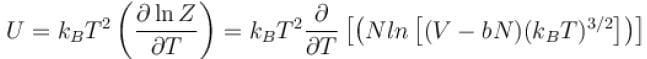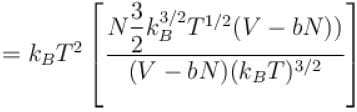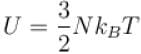## Topic wise Tests for IIT JAM Physics

217 tests
 Use Code STAYHOME200 and get INR 200 additional OFF Use Coupon Code
Information about Thermodynamic Potential NAT Level – 2 Page
In this test you can find the Exam questions for Thermodynamic Potential NAT Level – 2 solved & explained in the simplest way possible. Besides giving Questions and answers for Thermodynamic Potential NAT Level – 2, EduRev gives you an ample number of Online tests for practice

217 tests